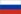Saint Petersburg, Russia

# Applied Mathematics

## Прикладная математика

Bachelor's
Language: RussianSubject area: mathematics and statistics
University website: www.smtu.ru
Applied Mathematics
Applied mathematics is the application of mathematical methods by different fields such as science, engineering, business, computer science, and industry. Thus, applied mathematics is a combination of mathematical science and specialized knowledge. The term "applied mathematics" also describes the professional specialty in which mathematicians work on practical problems by formulating and studying mathematical models. In the past, practical applications have motivated the development of mathematical theories, which then became the subject of study in pure mathematics where abstract concepts are studied for their own sake. The activity of applied mathematics is thus intimately connected with research in pure mathematics.
Mathematics
Mathematics (from Greek μάθημα máthēma, "knowledge, study, learning") is the study of such topics as quantity, structure, space, and change. It has no generally accepted definition.
Mathematics
Mathematics is a versatile art; it can be applied to widely different purposes. Math has no morality; it does not care what it counts or what it proves.
Brian Stableford, Ashes and Tombstones, in Peter Crowther (ed.) Moon Shots (1999), reprinted in David G. Hartwell (ed.) Year's Best SF 5 (2000), p. 412
Mathematics
Archimedes will be remembered when Aeschylus is forgotten, because languages die and mathematical ideas do not. "Immortality" may be a silly word, but probably a mathematician has the best chance of whatever it may mean.
G. H. Hardy, A Mathematician's Apology (London 1941).. Quotations by Hardy. Gap.dcs.st-and.ac.uk. Retrieved on 27 November 2013..
Mathematics
Mathematical development in England was at a low ebb in the early decades of the nineteenth century, with Cambridge stagnating in the shadow of Newton, who had produced his mathematics nearly a century and a half earlier. This dead hand of tradition, which stifled much initiative and originality, was in sharp contrast to the situation in France.
D. Mary Cannell, "George Green Mathematician and Physicist 1793-1841: The background to his life and work" p. xxviii (second edition, 2001).